Mathematics (Math) is the study of physical properties and measurable change, logic is a philosophy that uses mathematics-based reasoning. Logic can also apply non-mathematics-based reasoning (see section on logic in philosophy for all non-mathematics based logic).

## Einstein Wrote ‘E=mc^2’ in His Famous Paper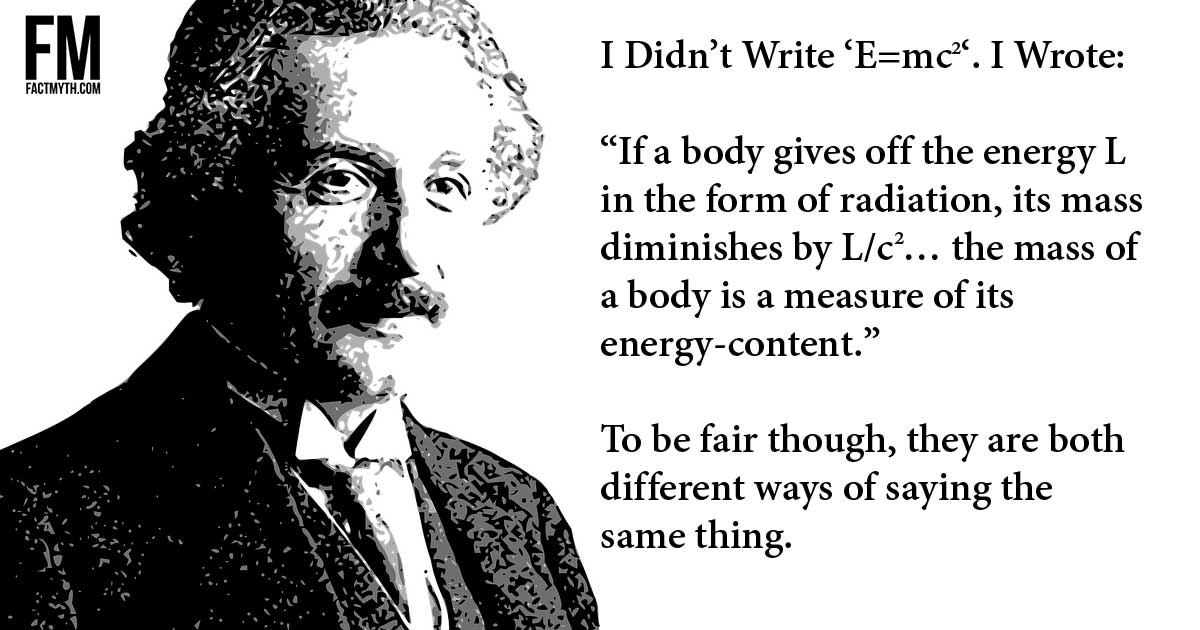Einstein’s 1905 paper on mass-energy equivalence doesn’t actually say ‘E=mc^2‘ it says, roughly, ‘m=L/c^2‘.

## A System is a Set of Properties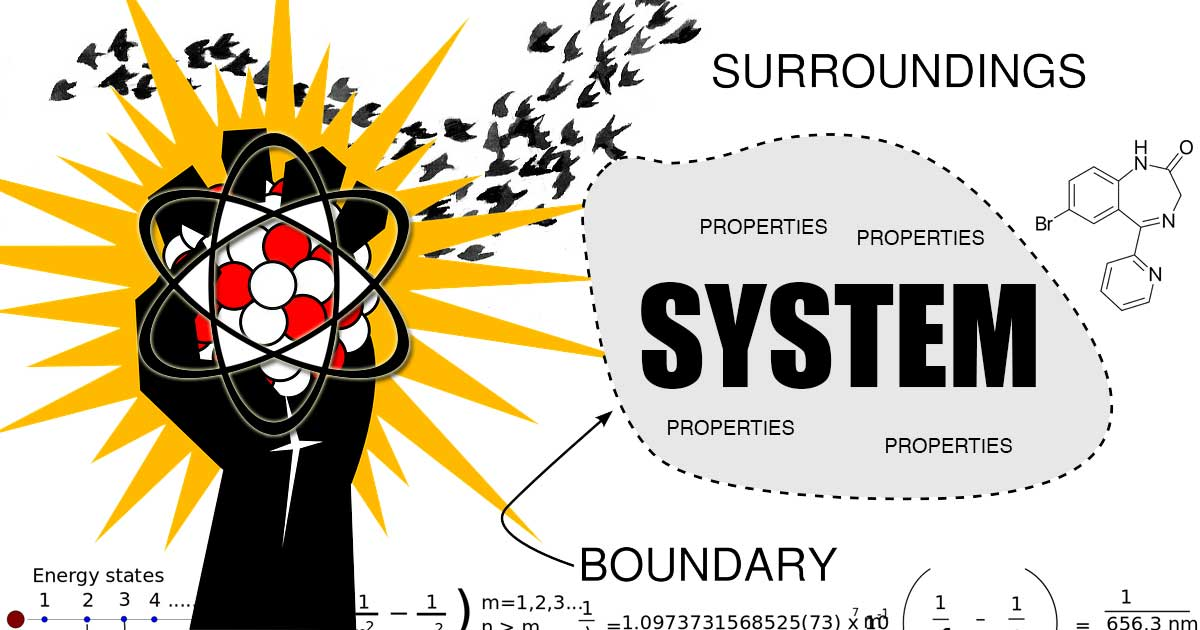A system is any “bound”, finite, set of physical and/or conceptual properties (elements) such as physical objects, rules, or space time coordinates.

## A Small Part of a Curved Surface Will Look “Flat”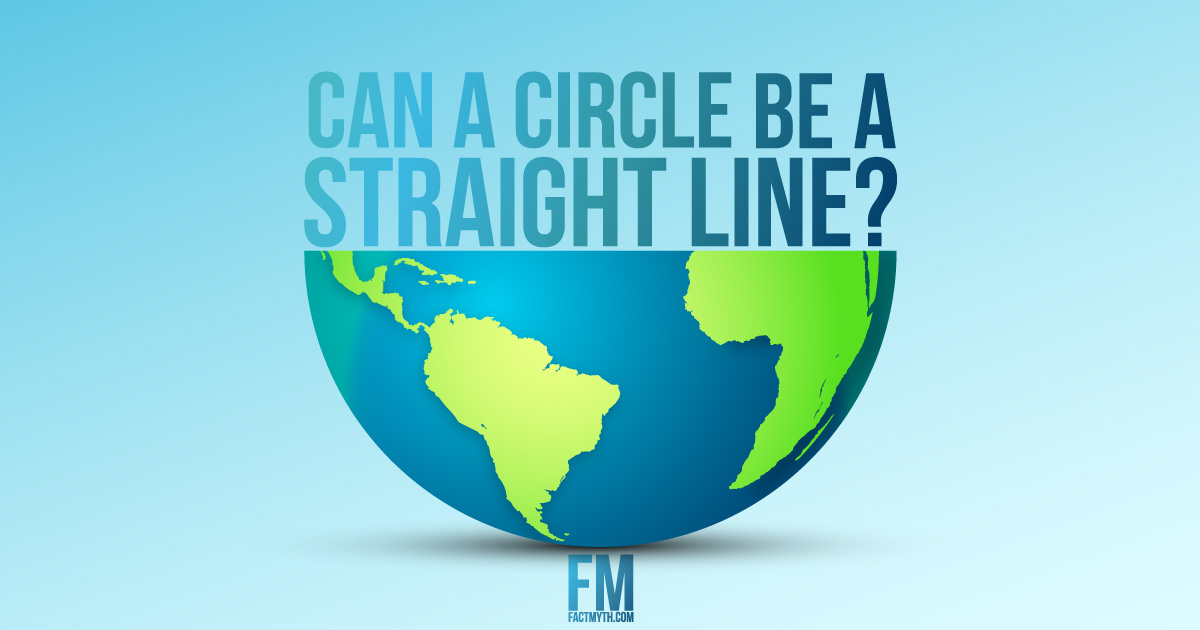If you look at a curved surface from close up it will look “flat”, if you change your perspective and “zoom out” it will look “round”.

## Conway’s Game of Life Models Evolution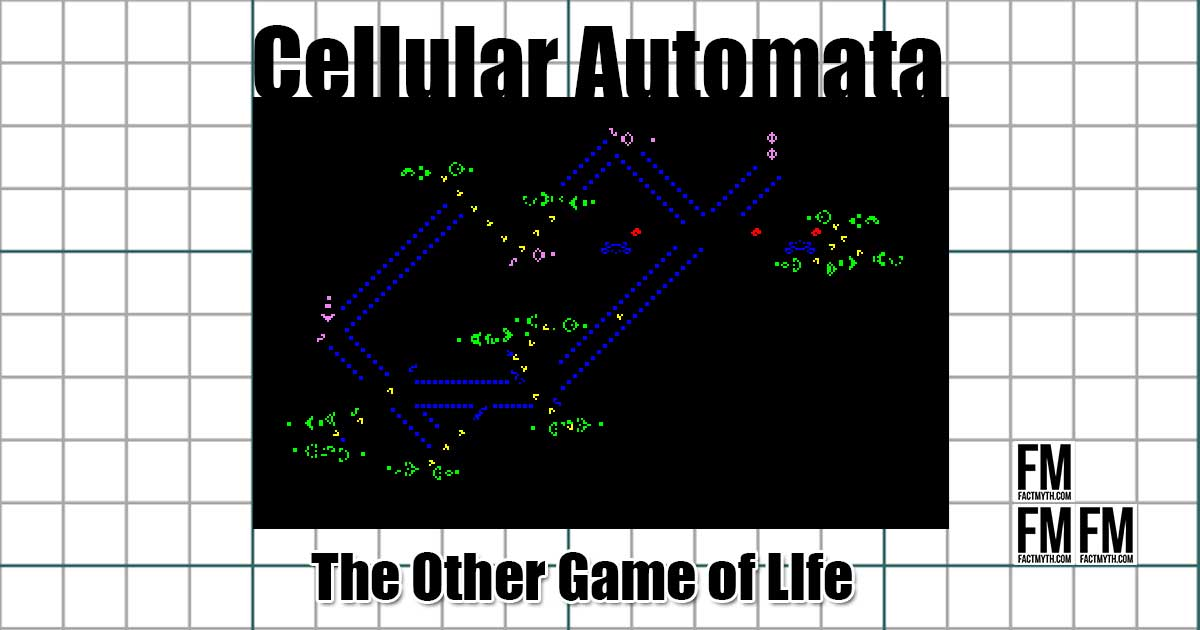Conway’s Game of Life is a simple rule-set that models the evolution of systems. It’s a “zero-player” computer program that demonstrates “cellular automata”.

## Everything Depends on Frame of Reference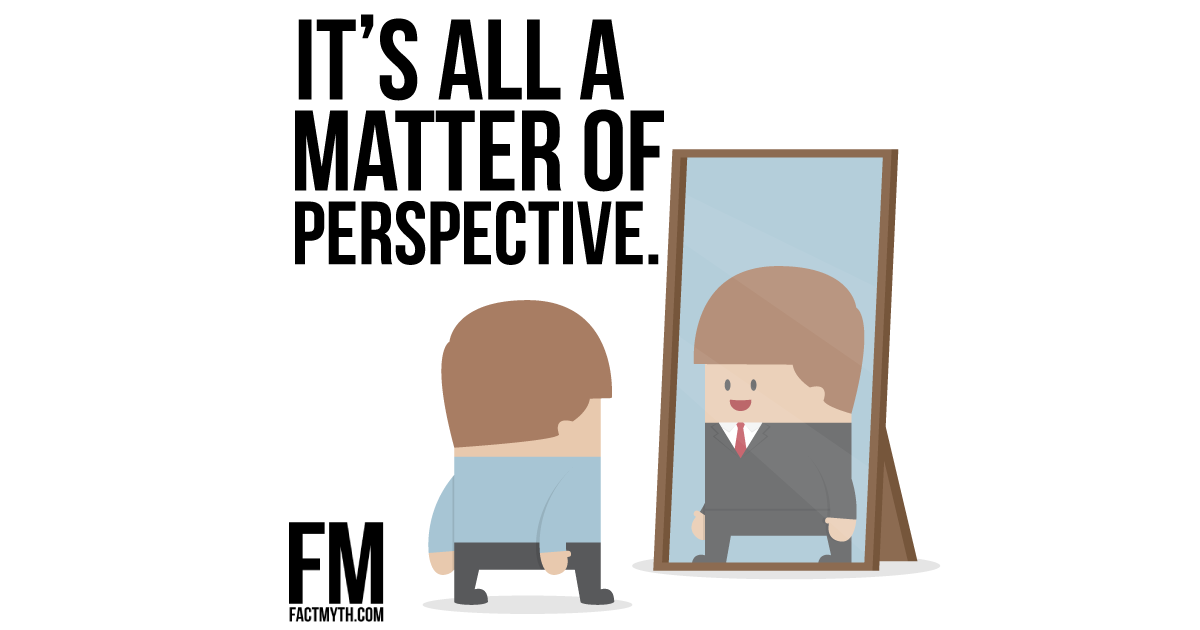Everything we perceive depends on our frame of reference. What we observe is relative to our point of view. In other words, “it is all a matter of perspective”.

## Infinity is a Number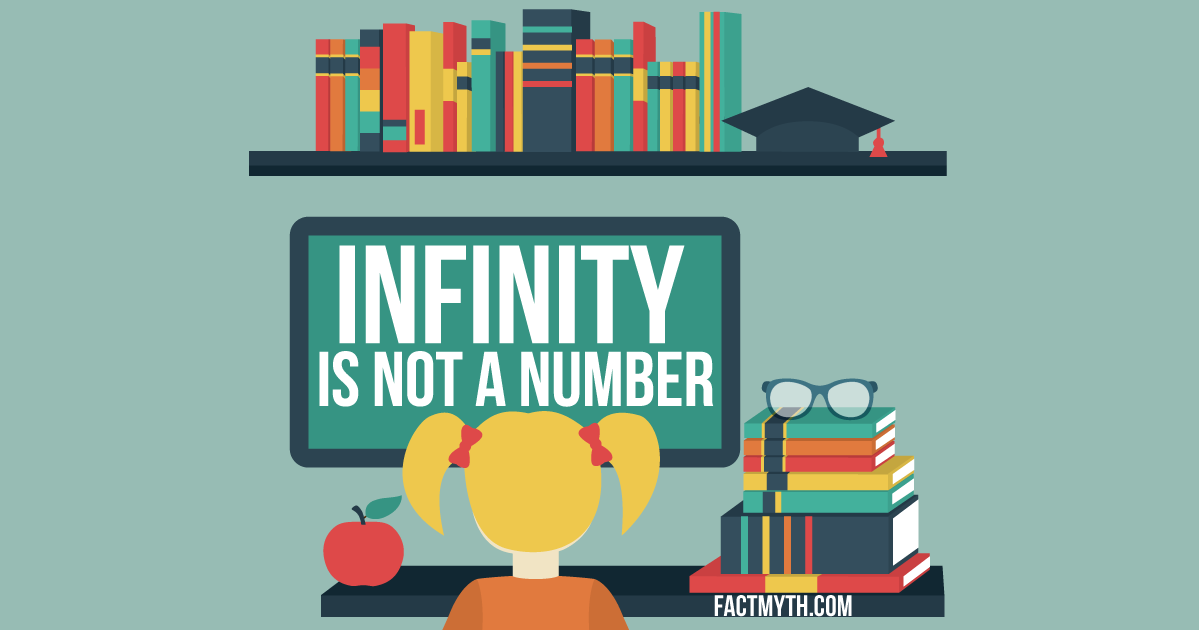Infinity (∞) isn’t a number, it’s a concept. It describes something that can’t be expressed by a “finite” natural or real number.

## There are Different Types of InfinityThere are different types of infinity (∞) which differ by size, countability, “flavor” and more. Most types have practical real world applications.

## Physics is Different than Metaphysics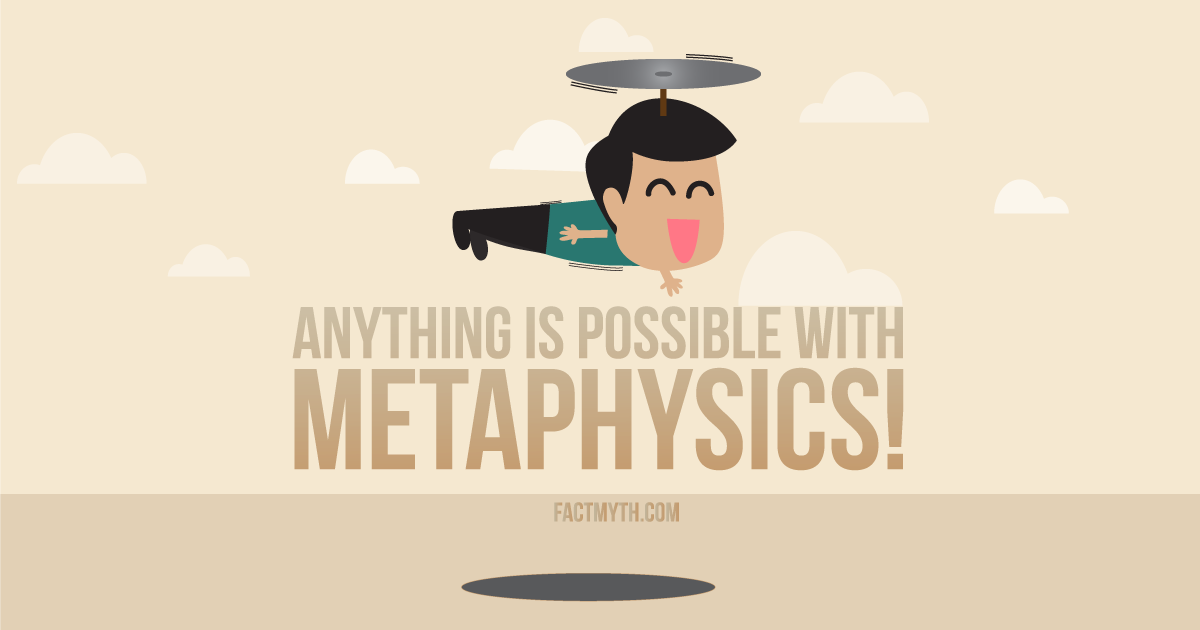Physics is a branch of physical science rooted in math that asks “how does it work?” Metaphysics is a broad branch of philosophy that asks, “What is its true nature?”

## The Earth Isn’t FlatThe Earth is not flat; the Earth is an oblate spheroid (a bumpy sphere with a fat equator and skinny poles). There are many ways to prove the earth’s geometry.

## E=mc^2 (Mass and Energy are Equivalent)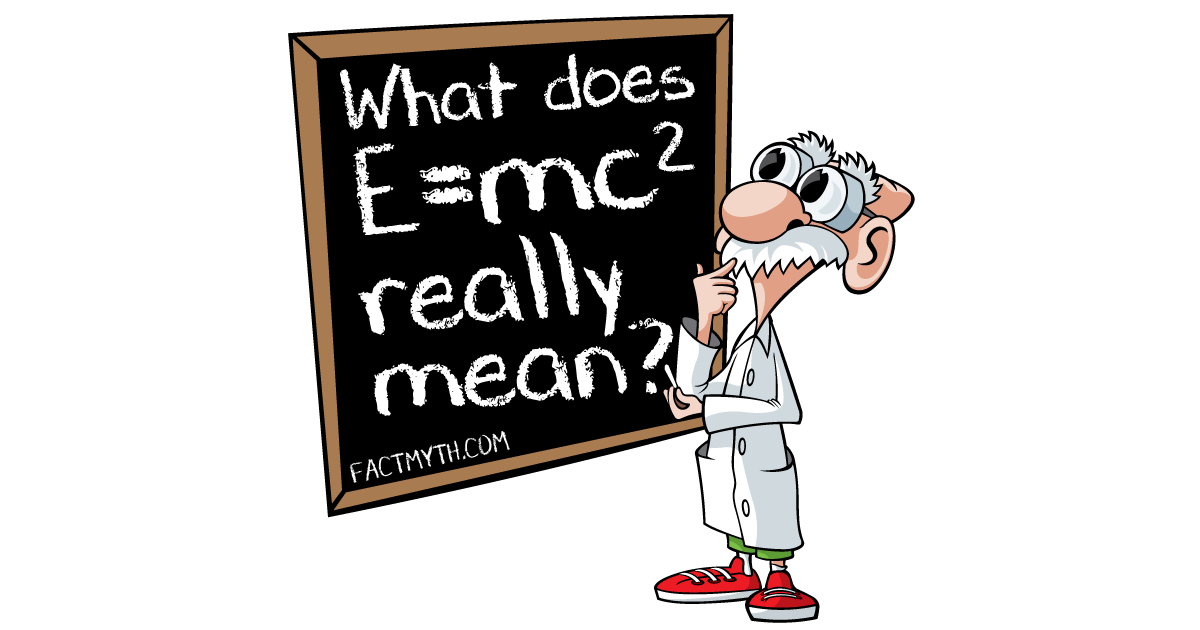Einstein’s mass-energy equivalence equation (E=mc2) shows that mass and energy are equivalent (but not “exactly the same”) properties of a physical system.